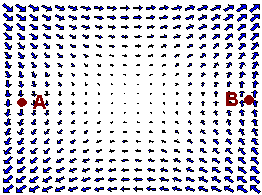$$\newcommand{\id}{\mathrm{id}}$$ $$\newcommand{\Span}{\mathrm{span}}$$ $$\newcommand{\kernel}{\mathrm{null}\,}$$ $$\newcommand{\range}{\mathrm{range}\,}$$ $$\newcommand{\RealPart}{\mathrm{Re}}$$ $$\newcommand{\ImaginaryPart}{\mathrm{Im}}$$ $$\newcommand{\Argument}{\mathrm{Arg}}$$ $$\newcommand{\norm}{\| #1 \|}$$ $$\newcommand{\inner}{\langle #1, #2 \rangle}$$ $$\newcommand{\Span}{\mathrm{span}}$$

# 4.4: Conservative Vector Fields and Independence of Path

$$\newcommand{\vecs}{\overset { \rightharpoonup} {\mathbf{#1}} }$$ $$\newcommand{\vecd}{\overset{-\!-\!\rightharpoonup}{\vphantom{a}\smash {#1}}}$$$$\newcommand{\id}{\mathrm{id}}$$ $$\newcommand{\Span}{\mathrm{span}}$$ $$\newcommand{\kernel}{\mathrm{null}\,}$$ $$\newcommand{\range}{\mathrm{range}\,}$$ $$\newcommand{\RealPart}{\mathrm{Re}}$$ $$\newcommand{\ImaginaryPart}{\mathrm{Im}}$$ $$\newcommand{\Argument}{\mathrm{Arg}}$$ $$\newcommand{\norm}{\| #1 \|}$$ $$\newcommand{\inner}{\langle #1, #2 \rangle}$$ $$\newcommand{\Span}{\mathrm{span}}$$ $$\newcommand{\id}{\mathrm{id}}$$ $$\newcommand{\Span}{\mathrm{span}}$$ $$\newcommand{\kernel}{\mathrm{null}\,}$$ $$\newcommand{\range}{\mathrm{range}\,}$$ $$\newcommand{\RealPart}{\mathrm{Re}}$$ $$\newcommand{\ImaginaryPart}{\mathrm{Im}}$$ $$\newcommand{\Argument}{\mathrm{Arg}}$$ $$\newcommand{\norm}{\| #1 \|}$$ $$\newcommand{\inner}{\langle #1, #2 \rangle}$$ $$\newcommand{\Span}{\mathrm{span}}$$

## The Fundamental Theorem of Line Integrals

Consider the force field representing the wind shown belowYou are a pilot attempting to minimize the work your engines need to do. Does it matter which path you take? Clearly the red path goes with the wind and the green path goes against the wind. With this vector field, work is dependent on the path that is taken.

Next consider the vector field

$\textbf{F}(x,y) = y\hat{\textbf{i}} + x \hat{\textbf{j}} \nonumber$

shown belowIt turns out that going from point A to point B, every path leads to the same amount of work done. What is special about this vector field?

The key here, as you can quickly check, is that the vector field $$\textbf{F}$$ is conservative with $$M_y = N_x$$. Since for a conservative vector field, all paths produce the same amount of work, we seek a formula that gives this work quantity. The theorem below shows us how to find this quantity. Notice the strong resemblance to the fundamental theorem of calculus.

Theorem: The Fundamental Theorem of Line Integrals

Let $$\textbf{F}$$ be a conservative vector field with potential function $$f$$, and $$C$$ be any smooth curve starting at the point $$A$$ and ending at the point $$B$$. Then

$\int_C F \cdot dr = f(B)-f(A) \label{FTLI}$

Proof

To prove the fundamental theorem of line integrals we will use the following outcome of the chain rule:

If

$\bar{\textbf{r}}(t) = x(t) \hat{\textbf{i}} + y(t) \hat{\textbf{j}} \nonumber$

is a vector valued function, then

$\dfrac{d}{dt} f(\bar{\textbf{r}}(t)) = f_x x'(t) + f_y y'(t). \nonumber$

We are now ready to prove the theorem. We have

\begin{align*} \int_C \textbf{F} \cdot d \hat{\textbf{r}} &= \int_{a}^{b} \textbf{F}(x,y) \cdot \bar{\textbf{r}}' (t) \; dt \\[4pt] &= \int_{a}^{b} \big( f_x (x,y) \hat{\textbf{i}} + f_y(x,y) \hat{\textbf{j}} \big) \cdot \big( x'(t) \hat{\textbf{i}} + y'(t) \hat{\textbf{j}} \big) \; dt \\[4pt] &= \int_{a}^{b} \big( f_x (x,y)x'(t) + f_y (x,y)y'(t) \big) \; dt \\[4pt] &= \int_{a}^{b} \dfrac{d}{dt} \big(f(x(t), y(t)) \big)\; dt \\[4pt] &= f(x(b),y(b))-f(x(a),y(a)) \\[4pt] &=f(B) -f(A) \end{align*}

$$\square$$

The next example demonstrates the power of this theorem.

Example $$\PageIndex{1}$$

Find the work done by the vector field

$\textbf{F}(x,y) = (2x -3y) \hat{\textbf{i}} + (3y^2 - 3x) \hat{\textbf{j}} \nonumber$

along the curve indicated in the graph below.Solution

First notice that

$M_y = -3 = N_x \nonumber$

We can use the fundamental theorem of line integrals to solve this. There are two approaches.

Approach 1

We find the potential function. We have

$f_x = 2x - 3y \nonumber$

Integrating we get

$f(x,y) = x^2 - 3xy + c(y)\nonumber$

Now take the derivative with respect to $$y$$ to get

$f_y = -3x + c'(y) = 3y^2 - 3x. \nonumber$

Hence

$c'(y) = 3y^2 \nonumber$

and

$c(y) = y^3.\nonumber$

The potential function is

$f(x,y) = x^2 - 3xy + y^3.\nonumber$

Now use the fundamental theorem of line integrals (Equation \ref{FTLI} to get

$f(B) - f(A) = f(1,0) - f(0,0) = 1.\nonumber$

Approach 2

Since the vector field is conservative, any path from point A to point B will produce the same work. Hence the work over the easier line segment from $$(0,0)$$ to $$(1,0)$$ will also give the correct answer. We parameterize by

$\bar{r}(t)= t \hat{\text{i}} \;\;\;\; 0 \leq t \leq 1.\nonumber$

We have

$\bar{r}i(t) = \hat{\text{i}} \nonumber$

so that

\begin{align*} \textbf{F} \cdot d \hat{r} &= \big((2x-3y)\hat{\text{i}} + (3y^2-3x)\hat{\text{j}} \big)\ \cdot \hat{\text{i}} \\[4pt] &= 2x-3y \\[4pt] &= 2t . \end{align*} \nonumber

Now just integrate

$\int_0^1 2t \; dt = t^2 |_0^1 = 1. \nonumber$

## Independence of Path and Closed Curves

Example $$\PageIndex{2}$$

Find the work done by the vector field

$\textbf{F}(x,y) = (\cos x + y) \hat{\textbf{i}} + (x+e^{\sin y})\hat{\textbf{j}} + (\sin(\cos z)) \hat{\textbf{k}} \nonumber$

along the closed curve shown below.Solution

First we check that F is conservative. We have

\begin{align*} \text{Curl } \textbf{F} &= \begin{vmatrix} \hat{\textbf{i}} & \hat{\textbf{j}} & \hat{\textbf{k}} \\[4pt] \partial x & \partial y & \partial z \\[4pt] \cos x+y & x+ e^{\sin y} & \sin (\cos z) \end{vmatrix} \\[4pt] &= (0-0) \hat{\textbf{i}} - (0-0) \hat{\textbf{j}} + (1-1) \hat{\textbf{k}} \end{align*}

Since the vector field is conservative, we can use the fundamental theorem of line integrals. Notice that the curve begins and ends at the same place. We do not even need to find the potential function, since whatever it is, say $$f$$, we have

$f(A) - f(A) = 0.\nonumber$

In general, the work done by a conservative vector field is zero along any closed curve. The converse is also true, which we state without proof.

Conservative Vector Fields and Closed Curves

Let $$\vec{F}$$ be a vector field with components that have continuous first order partial derivatives and let $$C$$ be a piecewise smooth curve. Then the following three statements are equivalent

1. $$\vec{F}$$ is conservative.
2. $$\int_C \textbf{F}\cdot dx\$$ is independent of path.
3. $$\int_C \textbf{F} \cdot dr = 0$$ for all closed curves $$C$$.